Home > ACC7 > Chapter cc38 > Lesson cc38.2.4 > Problem8-109

8-109.
1. Compute each product or quotient. Convert the final answer to scientific notation if necessary. Homework Help ✎

1. (3 × 102)(2 × 103)

2. (2.75 × 10−2)(2.5 × 108)

3.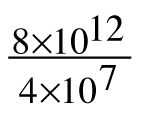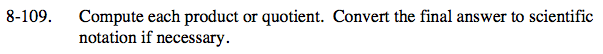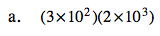You can also look at this problem as:

(3 · 2)(102 · 103)

6 · 105

Is this in scientific notation?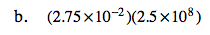See part (a).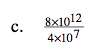You can also look at this problem as:

$\left(\frac{8}{4}\right)\left(\frac{10^{12}}{10^{7}}\right)$

2 · 105

Is this in scientific notation?